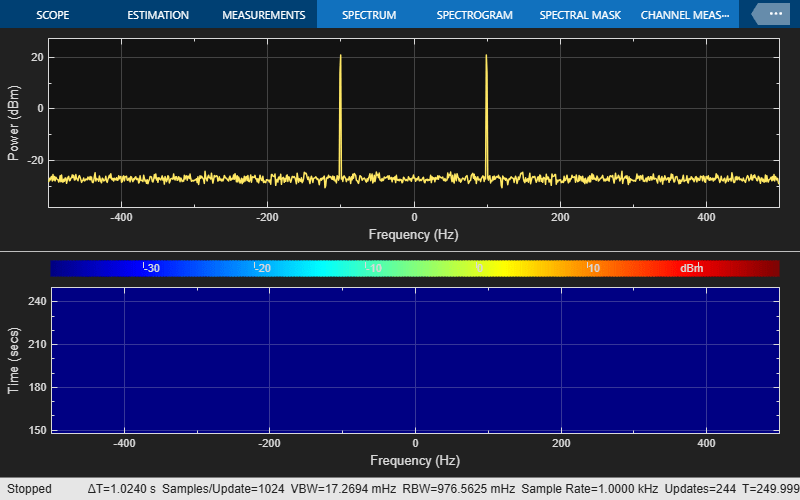Check spectrum analyzer for new data

## Syntax

``flag = isNewDataReady(scope)``

## Description

example

````flag = isNewDataReady(scope)` indicates whether the spectrum analyzer `scope` displays new spectral estimates. When logging spectrum analyzer data from the `spectrumAnalyzer` scope, use this function to ignore duplicate spectra from the `getSpectrumData` function.```

## Examples

collapse all

Generate a discrete sine wave. Then display the sine wave signal using the Spectrum Analyzer. While the Spectrum Analyzer is running, save the spectrum data to a table. The Spectrum Analyzer does not update at every time step. To avoid saving that redundant spectrum data, use the `isNewDataReady` function.

```wave = dsp.SineWave(Frequency=100,SampleRate=1000); wave.SamplesPerFrame = 1000; scope = spectrumAnalyzer(SampleRate=wave.SampleRate,... ViewType="spectrum-and-spectrogram"); data = []; for ii = 1:250 x = wave() + 0.05*randn(1000,1); scope(x); if scope.isNewDataReady data = [data;getSpectrumData(scope)]; end end release(scope); ```Show the first five rows in the table. You can see gaps in the simulation time in the `data` table. The missing rows indicate times where the Spectrum Analyzer was waiting for additional samples to update the spectrum. The `isNewDataReady` function prevented the script from saving that redundant data.

```data(1:5,:) ```
```ans = 5x4 table SimulationTime Spectrum Spectrogram FrequencyVector ______________ _______________ _________________ _______________ {[1.9990]} {1024x1 double} {100x1024 double} {1024x1 double} {[2.9990]} {1024x1 double} {100x1024 double} {1024x1 double} {[3.9990]} {1024x1 double} {100x1024 double} {1024x1 double} {[4.9990]} {1024x1 double} {100x1024 double} {1024x1 double} {[5.9990]} {1024x1 double} {100x1024 double} {1024x1 double} ```

## Input Arguments

collapse all

Spectrum analyzer from which you want to save data, specified as a `spectrumAnalyzer` object.

## Output Arguments

collapse all

Flag indicating new data, returned as one of these:

• `true` –– The Spectrum Analyzer shows new data.

• `false` –– The Spectrum Analyzer shows the same spectrum and no new data is available.

## Version History

Introduced in R2017b

expand all